NEET  >  Test: Dimensional Analysis & Its Applications (NCERT)

# Test: Dimensional Analysis & Its Applications (NCERT) - NEET

Test Description

## 10 Questions MCQ Test Physics Class 11 - Test: Dimensional Analysis & Its Applications (NCERT)

Test: Dimensional Analysis & Its Applications (NCERT) for NEET 2023 is part of Physics Class 11 preparation. The Test: Dimensional Analysis & Its Applications (NCERT) questions and answers have been prepared according to the NEET exam syllabus.The Test: Dimensional Analysis & Its Applications (NCERT) MCQs are made for NEET 2023 Exam. Find important definitions, questions, notes, meanings, examples, exercises, MCQs and online tests for Test: Dimensional Analysis & Its Applications (NCERT) below.
Solutions of Test: Dimensional Analysis & Its Applications (NCERT) questions in English are available as part of our Physics Class 11 for NEET & Test: Dimensional Analysis & Its Applications (NCERT) solutions in Hindi for Physics Class 11 course. Download more important topics, notes, lectures and mock test series for NEET Exam by signing up for free. Attempt Test: Dimensional Analysis & Its Applications (NCERT) | 10 questions in 10 minutes | Mock test for NEET preparation | Free important questions MCQ to study Physics Class 11 for NEET Exam | Download free PDF with solutions
 1 Crore+ students have signed up on EduRev. Have you?
Test: Dimensional Analysis & Its Applications (NCERT) - Question 1

### Checking the correctness of equations using the method of dimensions is based on

Detailed Solution for Test: Dimensional Analysis & Its Applications (NCERT) - Question 1

Only the dimensional accuracy can be checked with the help of principle of homogeneity of dimensions. This is limitation of principle of homogeneity of dimensions.

Test: Dimensional Analysis & Its Applications (NCERT) - Question 2

### Using the principle of homogeneity of dimensions, which of the following is correct?

Detailed Solution for Test: Dimensional Analysis & Its Applications (NCERT) - Question 2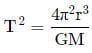Taking dimensions on both sides, we get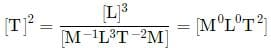∴ LHS = RHS
Now, T2 = 4π2r2
Taking dimensions on both sides
[T]2 = [L]2
∴ LHS ≠ RHS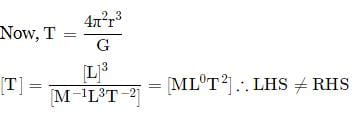Test: Dimensional Analysis & Its Applications (NCERT) - Question 3

### Which of the following relations is dimensionally incorrect?

Detailed Solution for Test: Dimensional Analysis & Its Applications (NCERT) - Question 3

As, we know, E = mc2 and energy released can be given as:
E = 931MeV
for, m = 1u
1u = 931.5MeV/c2 - Dimensionally correct [Dimension of mass]
1u = 1.67 × 10−27 kg - Dimensionally correct [Dimension of mass]
but, 931.5 MeV is having the dimension of energy so it can't be equated to dimension of mass or dimension of 1u.
Hence, dimensionally correct data is given in option(A)

Test: Dimensional Analysis & Its Applications (NCERT) - Question 4

Which of the following relations for the displacement of a particle undergoing simple harmonic motion is not correct dimensionally?

Detailed Solution for Test: Dimensional Analysis & Its Applications (NCERT) - Question 4

Dimensions on RHS must be displacement [L].
Arguments of sine and cosine are dimensionless. Hence, Option (c) is not correct.

Test: Dimensional Analysis & Its Applications (NCERT) - Question 5

The displacement of a progressive wave is represented by y = A sin(ωt - kx) where x is distance and t is time. The dimensions of ω/k are same as those of the

Detailed Solution for Test: Dimensional Analysis & Its Applications (NCERT) - Question 5

y = Asin(ωt − kx)
As (ωt − kx) represents an angle which is dimensionless, therefore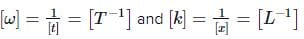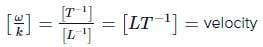Test: Dimensional Analysis & Its Applications (NCERT) - Question 6

If velocity of light c, Planck's constant h and gravitational constant G are taken as fundamental quantities then the dimensions of length will be:

Detailed Solution for Test: Dimensional Analysis & Its Applications (NCERT) - Question 6

Let l ∝ cxhy Gz ;l = kcx hy Gz
where k is a dimensionless constant and x, y and z are the exponents.
Equating dimensions on both sides, we get
[M0 LT0] =[LT−1] x [MLT−1] y [M−1 L3 T−2]z
= [My−z Lx+2y+3z T −x−y−2z]
Applying the principle of homogeneity of dimensions, we get
y − z = 0             ....(i)
x + 2y + 3z = 1     ....(ii)
− x − y − 2z = 0     ...(iii)
On solving Eqs. (i), (ii) and (iii), we get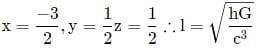Test: Dimensional Analysis & Its Applications (NCERT) - Question 7

A new system of units is proposed in which unit of mass is α kg, unit of length is β m and unit of time is γ s. What will be value of 5 J in this new system?

Detailed Solution for Test: Dimensional Analysis & Its Applications (NCERT) - Question 7

Joule is a unit of energy.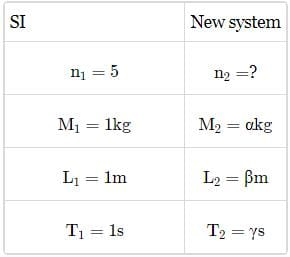Dimensional formula of energy is [ML2 T−2].
Comparing with [Ma Lb Tc], we get a = 1, b = 2,c = -2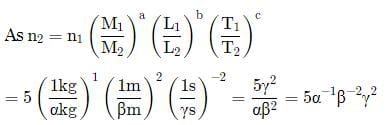Test: Dimensional Analysis & Its Applications (NCERT) - Question 8

If the energy, E = Gp hq cr where G is the universal gravitational constant, h is the Planck's constant and c is the velocity of light, then the values o f p, q and r are, respectively

Detailed Solution for Test: Dimensional Analysis & Its Applications (NCERT) - Question 8

E = Gp hcr…(i)
[M1L2T−2] = [M−1L3T−2]p[ML2T−1]q[LT−1]r
= [M−p+q L3p+2q+r T−2p−q−r]
Applying principle of homogeneity of dimensions, we get
−p + q = 1…(ii)
3p + 2q + r = 2…(iii)
−2p − q − r = −2…(iv)
we get p + q = 0…(v)
Adding (ii) and (v), we get q = 1/2
From (ii), we get p = q − 1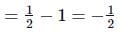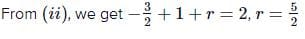Test: Dimensional Analysis & Its Applications (NCERT) - Question 9

The equation of state of a gas is given by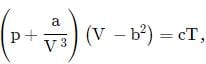where p,V,T are pressure, volume and temperature respectively and a,b,c are constants. The dimensions of a and b are respectively

Detailed Solution for Test: Dimensional Analysis & Its Applications (NCERT) - Question 9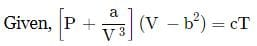Dimension of a/V3 = Dimensions of P
∴ Dimensions of a = dimensions of PV3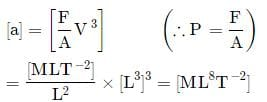Dimensions of b2  = dimensions of V
∴ [b] = [V]1/2 = [L3]1/2 or [b] = [L3/2]

Test: Dimensional Analysis & Its Applications (NCERT) - Question 10

The velocity of a particle (v) at an instant t is given by v = at + bt2. The dimension of b is the

Detailed Solution for Test: Dimensional Analysis & Its Applications (NCERT) - Question 10

v = at + bt2
[v] = [bt2] or [LT−1] = [bT2] or [b] = [LT−3]

## Physics Class 11

116 videos|451 docs|188 tests
Information about Test: Dimensional Analysis & Its Applications (NCERT) Page
In this test you can find the Exam questions for Test: Dimensional Analysis & Its Applications (NCERT) solved & explained in the simplest way possible. Besides giving Questions and answers for Test: Dimensional Analysis & Its Applications (NCERT), EduRev gives you an ample number of Online tests for practice

## Physics Class 11

116 videos|451 docs|188 tests

### How to Prepare for NEET

Read our guide to prepare for NEET which is created by Toppers & the best Teachers